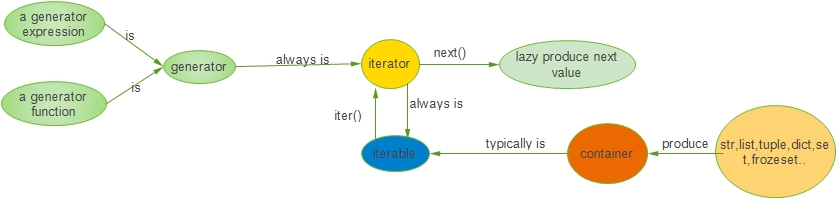﻿ Python之容器、迭代器、生成器

### 1.容器、可迭代对象、迭代器、生成器概念

1. 容器:存储许多元素的数据结构。通常存储在内存（迭代器、生成器是特例）可以使用in来判断某个元素是否在存在的对象都是容器

2.可迭代对象：可以使用iter()变成迭代器的对象都是可迭代对象,大部分容器都是可迭代对象(str,set,list,tuple,打开状态的files，sockets等等）

3.迭代器：它是一个带状态的对象，保存当前记录状态，当使用next()函数调用时，可以返回容器的下一个值。如果容器中没有更多元素了，则抛出StopIteration异常.

4.生成器：使用yield关键字，python都会把这个函数视为一个生成器.它返回了一个可迭代的对象，可以使用next()访问它里面的值了

5.如何使用迭代器呢？

1.方式是使用for 。。 in 。语法糖结构来遍历迭代器。
for默认的会调用__iter__返回一个迭代对象，然后调用next()方法调用迭代对象的__next__方法获取下一个值。

2.先获取迭代器对象，如使用it = iter(list)方法，在调用next(it)获取下一个值。最后没值了会抛出异常(StopIteration)### 2.具体实例来演示上面的内容

1.普通的函数实现

``````# 普通函数斐波那契数列
def fib1(n):
pre, cur = 0, 1
i = 0
while i < n:
print(cur, end=' ')
pre, cur = cur, cur + pre
i += 1

``````

2.普通的函数实现改进

``````# 普通函数斐波那契数列改进
def fib1(n):
result = []
pre, cur = 0, 1
i = 0
while i < n:
result.append(cur)
pre, cur = cur, cur + pre
i += 1
return result

print(lst)

``````

3.自定义一个可迭代的类来实现斐波那契数列

``````class Fib:

def __init__(self, stop):
self.stop = stop
self.pre = 0
self.cur = 1
self.start = 0
print('init finish')

def __iter__(self):
print('return iter')
return self

def __next__(self):
print('return next value')
if self.start < self.stop:
self.start += 1
x = self.cur
self.pre, self.cur = self.cur, self.cur + self.pre
return x
else:
raise StopIteration()

In : f = Fib(10)
Out:init finish

In : it = iter(f)
Out:return iter

1.它调用类中的__next__()方法
2.我们__next__()方法，返回当前值，并保存当前值记录状态和算出下一次调用要返回的值
In : next(it)
Out:return next value
Out: 1

In : next(it)
Out:return next value
Out: 2

``````

4.使用生成器

``````使用生成器，我们有两种形式：
1.创建生成器函数，使用yield关键字
2.使用生成器表达式
``````

4.1创建生成器函数

``````# 使用yield实现斐波那契数列
def fib(n):
pre, cur = 0, 1
i = 0
while i < n:
print('call this')
yield cur
pre, cur = cur, pre + cur
i += 1
print('here')

In : f = fib(10)
In : type(f)
Out: generator

In : next(f)
call this
Out: 1

In : next(f)
here
call this
Out: 2

In : next(f)
here
call this
Out: 3

``````

4.2生成器表达式实现斐波那契数列

``````# 从下面我们可以看出，使用()后a是一个generator类型
In : a = ( x for x in fib3(10))
In : type(a)
Out: generator

In : next(a)
call this
Out: 1

In : next(a)
here
call this
Out: 1

In : next(a)
here
call this
Out: 2

``````

5.对于可迭代对象遍历，我们一定会想到for循环

``````1.for循环的一般格式如下：
for iter_var in iterable:
suite_to_repeat

``````

``````class Fib:

def __init__(self, stop):
self.stop = stop
self.pre = 0
self.cur = 1
self.start = 0
print('init finish')

def __iter__(self):
print('return iter')
return self

def __next__(self):
print('return next value')
if self.start < self.stop:
self.start += 1
x = self.cur
self.pre, self.cur = self.cur, self.cur + self.pre
return x
else:
raise StopIteration()

def fortest():
for x in Fib(4):
print(x)

fortest()

import dis
dis.dis(fortest)

init finish
return iter
return next value
1
return next value
1
return next value
2
return next value
3

108           0 SETUP_LOOP              24 (to 26)
6 CALL_FUNCTION            1
8 GET_ITER
>>   10 FOR_ITER                12 (to 24)
12 STORE_FAST               0 (x)